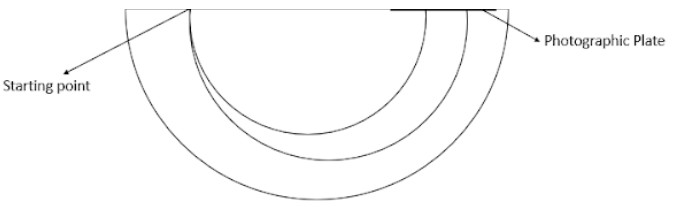QuestionAnswers

# The masses of two isotopes of chlorine are 34.980 and 36.978. If the radius of the circular path in Bainbridge spectrograph corresponding to lighter is 5 cm, the distance between the spots on photographic plate marked by the two isotopes will beA. 5.7 cmB. 0.57 cmC. 0.57 mmD. 0.57 mVerified
130.5k+ views
Hint: Inside a spectrograph, particles move at a particular speed in a plane perpendicular to a magnetic field. Due to the action of the magnetic field the articles will move in a circle, we can use the Lorentz force formula to find the radius in which those particles move.

Formula used:
Lorentz force
F = q(v×B)

\begin{align} & \dfrac{m{{v}^{2}}}{r}=qvB \\ & \Rightarrow r=\dfrac{mv}{qB} \\ \end{align}
\begin{align} & \dfrac{r}{5}=\dfrac{36.978}{34.980} \\ & \Rightarrow r=5.285 \\ \end{align}